# 无损压缩经典算法

### 1.进行文件压缩的必要性

1帧大小 = 1920 x 1080 x 3 = 6220800 bytes (1920x1080是每一帧的像素数，3指的是每个像素红绿蓝三个通道各占一个字节0~256)

***每秒大小 = 6220800 x 25 = 155.52MB!***(假设帧率为每秒25帧，很小了！)

90分钟：大约839.81GB

### 2.简单黑白图像的压缩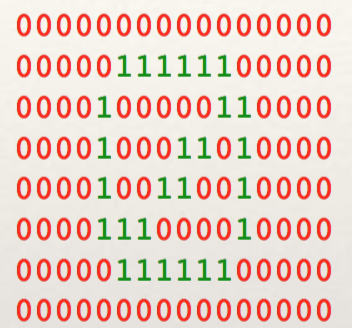21 6 9 1 5 2 8 1 3 2 1 1 8 1 2 2 2 1 8 6 21

### 4.信息的可压缩性-信息量和信息熵

#### 信息量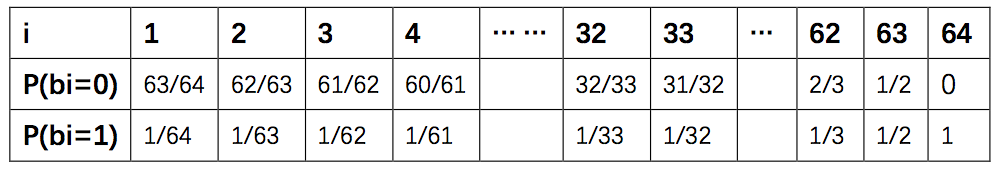h ( x ) = l o g 2 1 p ( x ) h(x)=log_2\frac{1}{p(x)}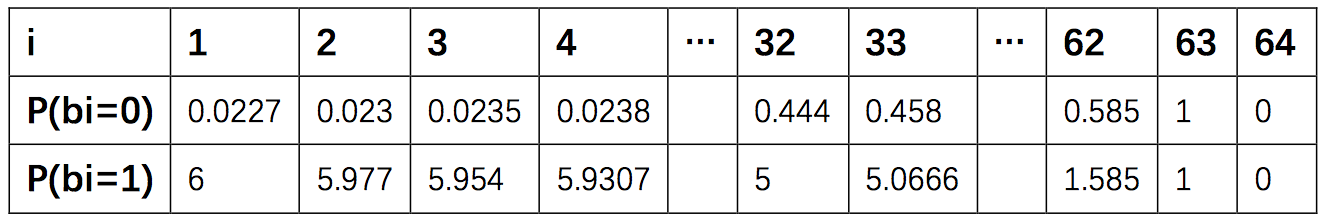Sum = 0.0227 + 0.023 + 0.0235 + 5.9307 = 6

∑ n = 1 i h ( b n ) = ( ∑ m = 66 − i 64 l o g 2 m m − 1 + l o g 2 1 1 / ( 65 − 1 ) ) = l o g 2 ( ( ∏ m = 66 − i 64 m m − 1 ) ( 65 − i ) ) = 6 \sum_{n=1}^ih(b_n)=(\sum_{m=66-i}^{64}log_2\frac{m}{m-1} +log_2\frac{1}{1/(65-1)}) = log_2((\prod_{m=66-i}^{64}\frac{m}{m-1}) (65-i)) = 6

#### 信息熵

H ( X ) = ∑ i P ( x i ) h ( x i ) H(X) = \sum_{i}P(x_i)h(x_i)

### 5.对编码算法的要求

1. 通过编码后的编码必须可以准确解码，编码必须确定地对应一种原码；
2. 编码算法得到的编码要容易解码，可以很容易的找到信息的末尾，可以在线解码，可以直接对编码进行解码而不用知道完整的编码信息；
3. 编码必须是压缩的，否则失去了编码的意义；

### 6.哈夫曼编码

a(0.25),b(0.25),c(0.2),d(0.15),e(0.15)

【如果不考虑编码算法，使用定长的编码来区别五个字母，不利用频率这些信息，那么五个字母每个字母需要用3bits表示(22=4,23=8).】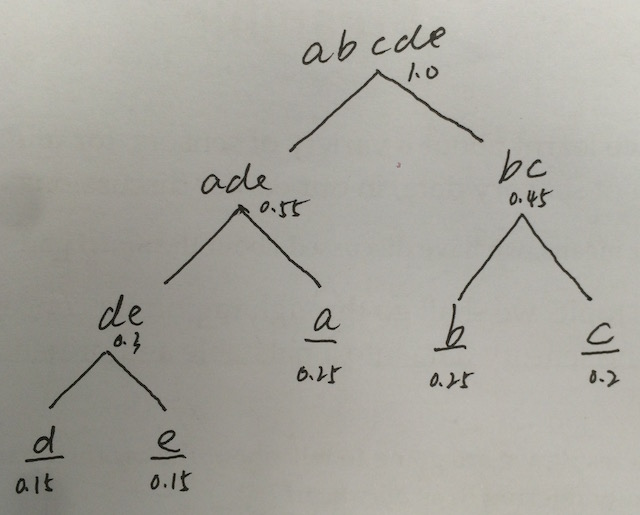#include <iostream>
using namespace std;

#define n 5         // 字符个数（叶子个数）
#define m 2*(n)-1   // 总节点数：1+2+4+8+...+n

typedef struct{
double weight;   // 节点权重
int lchild;      // 左子树
int rchild;      // 右子树
int parent;      // 父节点
}HTNODE;

typedef HTNODE HuffmanT[m];  // 一棵线性结构存储的哈弗曼树

/**
* 哈弗曼树初始化
*/
void InitHT(HuffmanT T)
{
for(int i=0; i<m; i++)
{
T[i].lchild=-1;
T[i].rchild=-1;
T[i].parent=-1;
}
// 依次输入每个节点的权重
for(int i=0; i<n; i++)
std::cin>>T[i].weight;
}

/**
* 找出还没有父节点的节点中权值最小的两个,p1和p2是要选出的权值最小的两个节点的下标，n1是新父节点的下标
*/
void SelectMin(HuffmanT T, int n1, int &p1, int &p2)
{
int i, j;
// 先任意找两个没有父节点的节点
for(i=0; i<n1; i++)
if(T[i].parent==-1)
{
p1=i;
break;
}
for(j=i+1; j<n1;j++)
if(T[j].parent==-1)
{
p2=j;
break;
}
// 搜索替换成权值最小的节点
for(i=0; i<n1; i++)
if((T[p1].weight>T[i].weight) && (T[i].parent==-1) && (p2!=i))
p1=i;
for(i=0; i<n1; i++)
if((T[p2].weight>T[i].weight) && (T[i].parent==-1) && (p1!=i))
p2=i;

}
/**
* 构造哈弗曼树
*/
void CreatHT(HuffmanT T)
{
int i, p1, p2;
InitHT(T);
// 非叶子节点
for(i=n; i<m; i++)
{
// 找出还没有父节点的节点中权值最小的两个
SelectMin(T, i, p1, p2);
T[p1].parent=T[p2].parent=i;
T[i].lchild=p1;
T[i].rchild=p2;
T[i].weight=T[p1].weight+T[p2].weight;
}
}

/**
* 打印哈弗曼树
*/
void printHT(HuffmanT T)
{
for(int i=0; i<m; i++)
{
std::cout<<T[i].weight<<'\t'<<T[i].parent<<'\t'<<T[i].rchild<<'\t'<<T[i].lchild<<std::endl;
}
}

/**
* 前台测试
*/
int main(){
HuffmanT T;
CreatHT(T);
printHT(T);
return 0;
}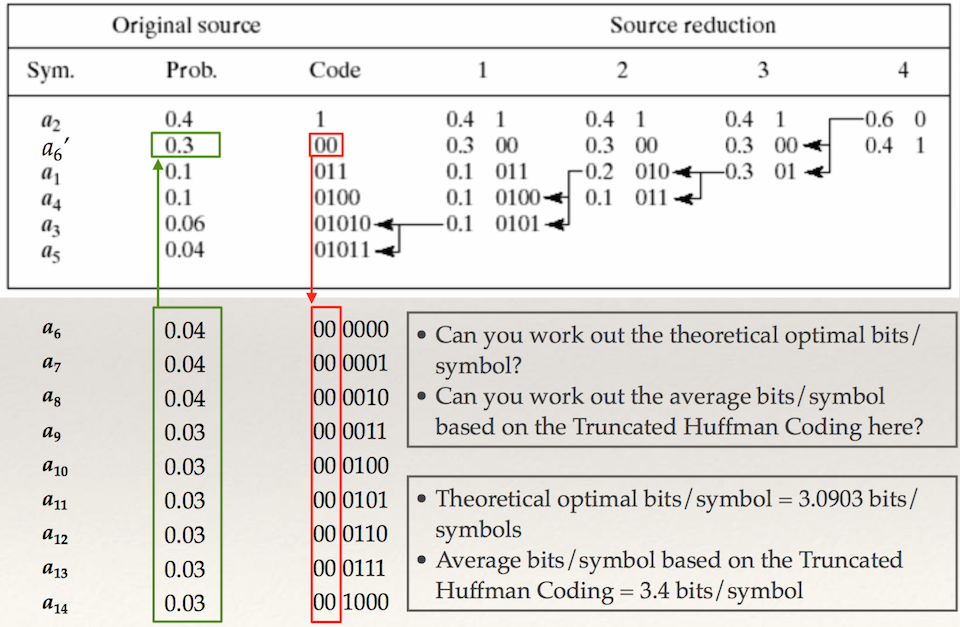Shift Coding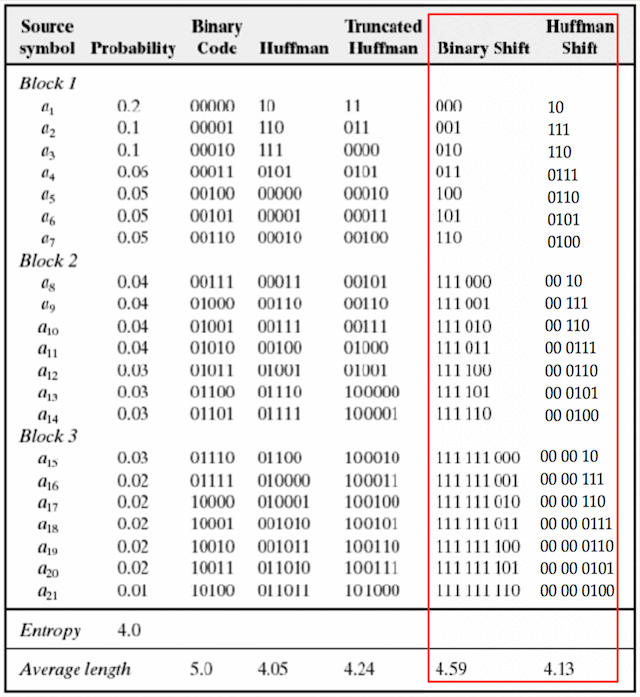### 7.Lempel-Ziv压缩算法

LZ算法及其衍生变形算法是压缩算法的一个系列。LZ77和LZ78算法分别在1977年和1978年被创造出来。虽然他们名字差不多，但是算法方法完全不同。这一系列算法主要适用于字母数量有限的信息，比如文字、源码等。流行的GIF和PNG格式的图像，使用颜色数量有限的颜色空间，其压缩就采用了两种算法的灵活变形应用。

#### LZ77：

LZ77压缩算法编码原理详解(结合图片和简单代码)

LZ77算法原理及实现

LZ77算法的思想是在编码解码过程中，使用之前刚结束编解码的部分数据的位置索引来代替当前要编解码的数据，压缩的实现靠的是之前编解码结束的部分数据和当前数据的重复性。

SB(search-buffer):刚过去的固定长度的数据缓冲，搜索缓冲区，也就是临时的数据字典，要从这里面搜索重复数据获得压缩索引；

Cursor:一个指针，指的是SB和LAB缓冲之间的边界处

**Token（p,l,c）**编码的结果：

• p: 第一个数字指的是SB中开始匹配的位置，注意是从Cursor往前倒着数，从1开始数
• l: 第二个数字指的是匹配成功的字符个数;
• c: 第三个指的是LAB中匹配结束的下一个字符;

***注意：***在匹配时是搜索字典中最长的匹配，且当前的LAB区域如果继续匹配也继续搜索（比如匹配到SB的最后一个字符后下一个到LAB的第一个字符仍然匹配则继续，直到不匹配或匹配数达到了LAB的长度限制则停止。如果在SB中一个都不匹配则不继续搜索，排除LAB第一个字符自身无尽循环匹配的情况）。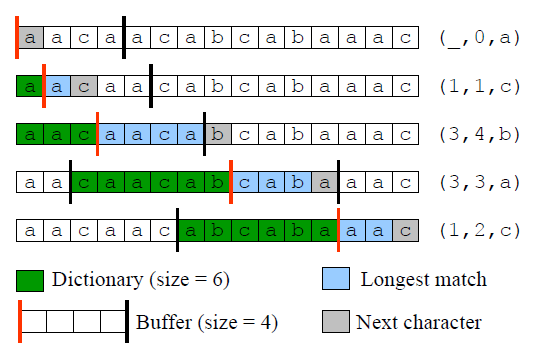#### LZ77的变形

***LZR：***就是SB搜索缓冲的长度不固定了，算法输出的token位长度也是可变的。

***LZH：***算法的输出结果又进行了哈夫曼编压缩。

***DEFLATE:***当前最流行的基于LZ77的压缩算法，是很多通用的Unix压缩项目‘gzip’的一部分。

#### LZ78:

***示例：***对字符串“abacbabaccbabbaca“进行LZ78编码。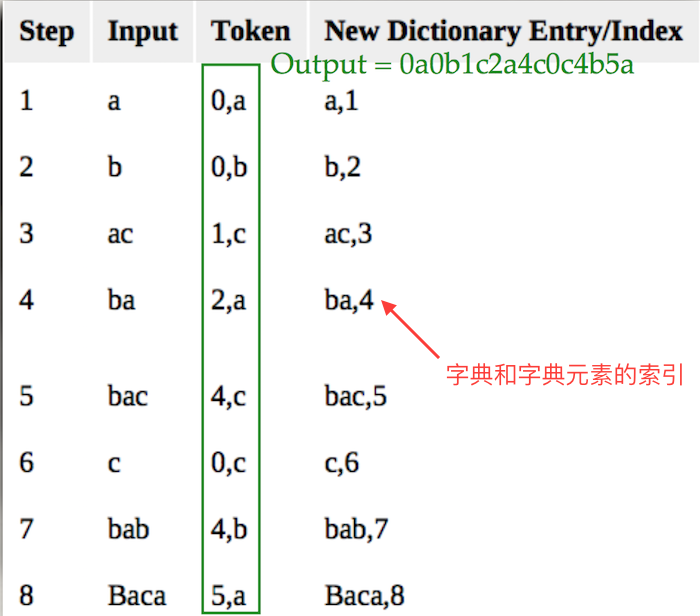#### LZ78的变形算法

LZC

LZW

***示例：***对字符串“abacbabaccbabbaca”进行LZW编码：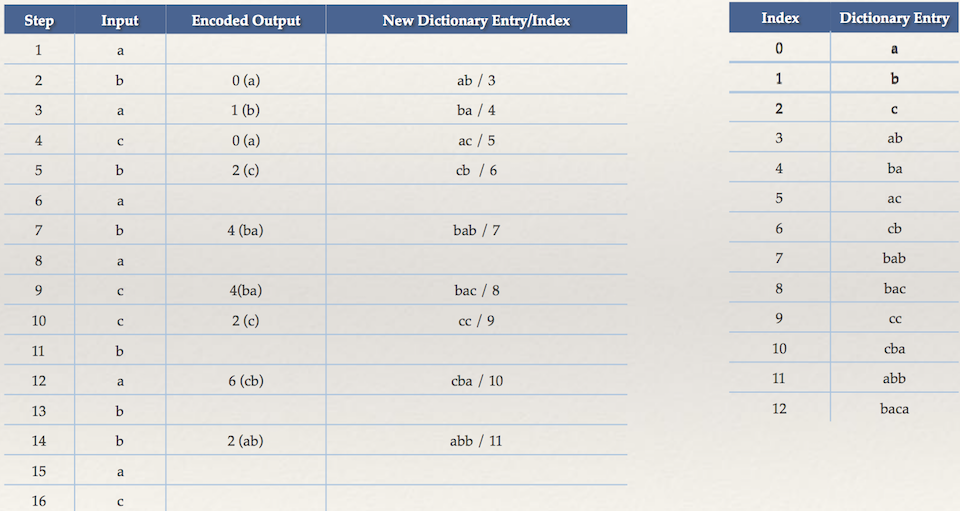### 算术编码

11-171万+09-202万+
09-23606
12-12218
08-22
07-302122
03-032万+
01-069976点击重新获取扫码支付余额充值